# Run Mandel’s h and k statistics to detect outliers

2017-10-20

This tutorial will help you compute and interpret Mandel’s h and k statistics for outliers in Excel using XLSTAT.

## Dataset for testing differences between samples with Mandel's statistics

An Excel sheet with both the data and the results can be downloaded by clicking here.

Four samples have been obtained from two different distributions: 3 from a normal distribution with mean 0 and variance 2 and one from a normal distribution with mean 0 and variance 5 .We suppose that each sample is related to the results of a laboratory. We wish to test if there are differences between laboratories' results.

## Goal of this tutorial

We would like to detect if means and variances are homogeneous using the h and k statistics of Mandel.

## Setting up a h and k Mandel's statistics analysis

To start the Mandel's h and k statistics go to the menu Testing outliers / Mandel's h and k statistcs.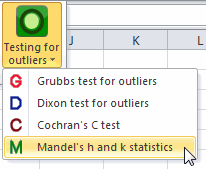In the General tab, select the data. Four columns should be selected with the option one column per group.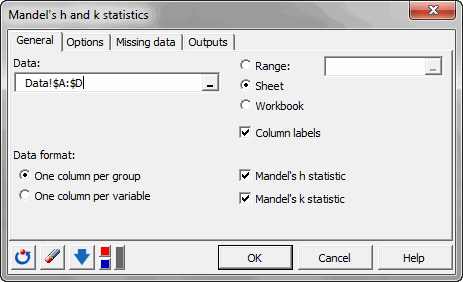## Results of a h and k Mandel's statistics

In the following table, we can see the h and k statistics for each laboratory.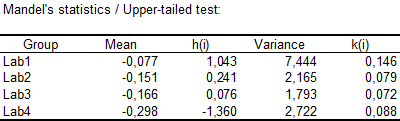In the following graphic, the h statistics are displayed together with the critical value. We can see that none of the values are outside the critical interval.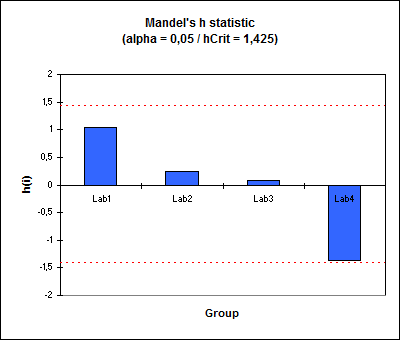The same graphic is available for the k statistic. It also shows that none of the laboratory obtain an outlying k statistic.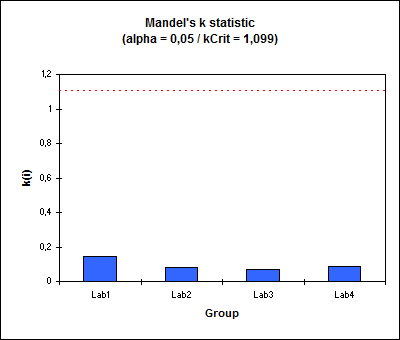We have shown that there is no outlying laboratory in this study.

#### Contact our technical support team: support@xlstat.com

https://cdn.desk.com/
false
desk